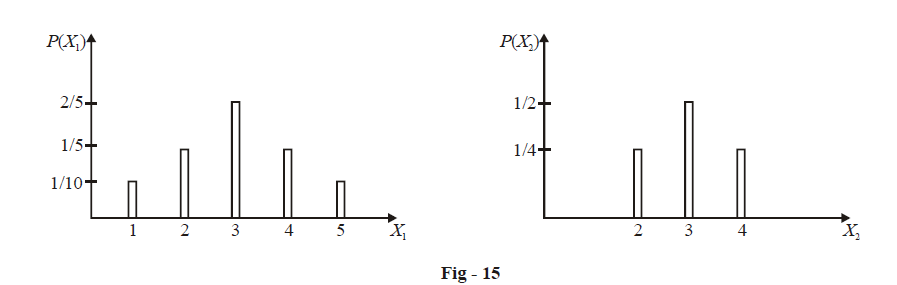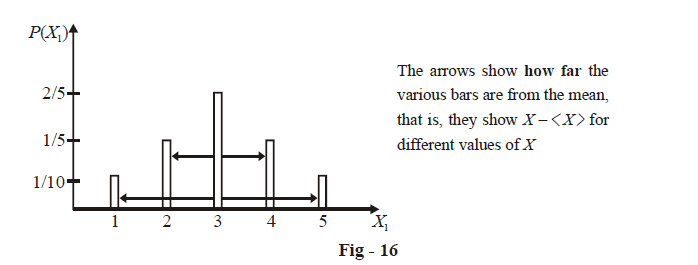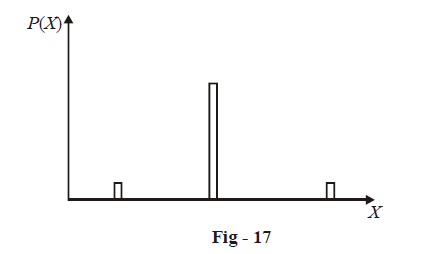# Mean and Variance

Go back to  'Probability'

It is in most cases very useful to talk about the mean of a random variable X. For example, in the experiment above of four tosses of a coin, someone might want to know the average number of heads obtained. Now the reader may wonder about what meaning to attach to the phrase “average number of heads”. After all, we are doing the experiment only once and we’ll obtain a particular value for the number of Heads, say 0 or 1 or 2 or 3 or 4, but what is then this “average number of heads”?

By the average number of heads we mean this: repeat the experiment an indefinitely large number of times. Each time you’ll get a certain number of Heads. Take the average of the number of Heads obtained in each repetition of the experiment. For example, if in 5 repetitions of this experiment, you obtain 2, 2, 3, 1, 1 Heads respectively, the average number of Heads would be (2 + 2 + 3 + 1 + 1) / 5 = 1.9. This is not a natural number, which shouldn’t worry you since it is an average over the 5 repetitions. To calculate the true average, you have to repeat the experiment an indefinitely large number of times.

An alert reader might have realized that the average value of a RV is easily calculable through its PD. For example, let us calculate the true average number of heads in the experiment of Example - 18. The PD is reproduced below:

 X 0 1 2 3 4 P(X) \begin{align}\frac{1}{16}\end{align} \begin{align}\frac{1}{4}\end{align} \begin{align}\frac{3}{8}\end{align} \begin{align}\frac{1}{4}\end{align} \begin{align}\frac{1}{16}\end{align}

Thus, for example,\begin{align}P(X=1)=\frac{1}{4}\end{align} , which means that if the experiment is repeated an indefinitely large number of times, we’ll obtain Heads exactly once, (about) \begin{align}{{\frac{1}{4}}^{th}}\end{align} of the time. Similarly, (about) \begin{align}{{\frac{3}{8}}^{th}}\end{align}of the time, Heads will be obtained exactly twice, and so on. Let us denote the number of repetition of the experiment by N, where $$N\to \infty$$, Thus, the average number of Heads per repetition would be (< > denotes average)

\begin{align}& <\text{Heads}>\ =\frac{\text{Total no}\text{. of Heads in N repetitions}}{\text{N}} \\ &\qquad\qquad\quad \;\;=\frac{0\times \frac{N}{16}+1\times \frac{N}{4}+2\times \frac{3N}{8}+3\times \frac{N}{4}+4\times \frac{N}{16}}{N} \\ & \qquad\qquad\quad \;\;=0\times \frac{1}{16}+1\times \frac{1}{4}+2\times \frac{3}{8}+3\times \frac{1}{4}+4\times \frac{1}{16} \\ & \qquad\qquad\quad\;=\sum \left( \text{Value of the RV} \right)\text{ }\times \text{ }\left( \text{Corresponding Probability of this value} \right) \\ \end{align}

Thus, we see that if a RV X has possible values x1, x2, ....xn with respective probabilities p1, p2, ...., pn, the mean of X, denote by $$\left\langle X \right\rangle$$ , is simply given by

$\left\langle X \right\rangle =\sum\limits_{i=1}^{n}{{{x}_{i}}\,{{p}_{i}}}\qquad\qquad\qquad.......\left( 1 \right)$

As another example, recall the experiment of rolling two dice where the RV X was the sum of the numbers on the two dice. The PD of X is given in the table on Page - 42, and the average value of X is

\begin{align}& \left\langle X \right\rangle \ =2\times \frac{1}{36}+3\times \frac{1}{18}+4\times \frac{1}{12}+5\times \frac{1}{9}+6\times \frac{5}{36}+7\times \frac{1}{6} \\ & \quad\qquad+8\times \frac{5}{36}+9\times \frac{1}{9}+10\times \frac{1}{12}+11\times \frac{1}{18}+12\times \frac{1}{36} \\ &\quad\quad =7 \\ \end{align}

The average value is also called the expected value, which signifies that it is what we can expect to obtain by averaging the RV’s value over a large number of repetitions of the experiment. Note that the value itself may not be expected in the general sense - the “expected value” itself may be unlikely or even impossible. For example, in the rolling of a fair die, the expected value of the number that shows up is 3.5 (verify), which in itself can never be a possible outcome. Thus, you must take care while interpreting the expected value - see it as an average of the RV’s values when the experiment is repeated indefinitely.

Another quantity of great significance associated with any RV X is its variance, denoted by Var(X). To understand this properly, consider two RVs X1 and X2 and their PDs shown in graphical form below.Both the RVs have an expected value of 3 (verify), but it is obvious that there is a significant difference between the two distributions. What is this difference? Can you put it into words? And more importantly, can you quantify it?

It turns out that we can, in a way very simple to understand. The ‘data’ or the PD of X1 is more widely spread than that of X2. This is what is obvious visually, but we must now assign a numerical value to this spread. So what we’ll do is measure the spread of the PD about the mean of the RV. For both X1 and X2, the mean is 3, but the PD of X1 is spread more about 3 than that of X2. We now quantity the spread in X1.Observe that the various value of  $$X-\left\langle X \right\rangle$$ tell us how far the corresponding values of X are from the mean (which is fixed). One way that may come to your mind to measure the spread is sum all these distances, i.e.

$'Spread'\text{ }={{\sum\limits_{\begin{smallmatrix} \text{For all} \\ \text{values } \\ \text{of }X \end{smallmatrix}}{\left(\text{X}-\left\langle X \right\rangle \right)}}}$

However, a little thinking should immediately make it obvious to you that the right hand side is always 0, because the data is spread in such a way around the mean that positive contributions to the sum from those X values greater than $$\left\langle X \right\rangle$$ and negative contributions from those X values smaller than  $$\left\langle X \right\rangle$$ exactly cancel out. Work it out yourself.

So what we do is use the sum of the squares of these distances:

$'Spread'\text{ }={{\sum\limits_{\begin{smallmatrix} \text{For all} \\ \text{values } \\ \text{of }X \end{smallmatrix}}{\left(\text{X}-\left\langle X \right\rangle \right)}}^{2}}$

However, there is still something missing. To understand what consider the following PD:Although the PD seems visually widespread here, the probabilities of those X values far from the mean are extremely low, which means that their contribution to the spread must take into account how probable they are and so on. This is simply accomplished by multiplying the value of  $${{\left( X-\left\langle X \right\rangle \right)}^{2}}$$ with the probability of the corresponding value of X.

Thus, if X can take the values x1, x2, ......., xn with probabilities p1, p2, ..., pn, the spread in the PD of X can be appropriately represented by

$Spread\text{ }={{\sum\limits_{i\ =\ 1}^{n}{\left( {{x}_{i}}-\left\langle X \right\rangle \right)}}^{2}}{{p}_{i}}$

This definition of spread is termed the variance of X, and is denoted by Var(X). Statisticians defined another quantify for spread, called the standard deviation, denote by $$\sigma _{x}^{2}$$ , and related to the variance by

$Var(X)=\sigma _{X}^{2}$

Note that the expected value of X was

$\left\langle X \right\rangle =\ \sum\limits_{i=1}^{n}{{{x}_{i}}{{p}_{i}}}$

Similarly, variance is nothing but the expected value of  $${{({{x}_{i}}-\left\langle X \right\rangle )}^{2}}$$

$Var\left( X \right)=\left\langle {{\left( {{x}_{i}}-\left\langle X \right\rangle \right)}^{2}} \right\rangle \ =\ \sum\limits_{i=1}^{n}{{{\left( {{x}_{i}}-\left\langle X \right\rangle \right)}^{2}}{{p}_{i}}}$

Coming back to Fig-16, the variance in X1 is

\begin{align}& Var({{X}_{1}})=\ {{(1-3)}^{2}}\cdot \frac{1}{10}+{{(2-3)}^{2}}\cdot \frac{1}{5}+{{(3-3)}^{2}}\cdot \frac{2}{5} \\ &\qquad\qquad +{{(4-3)}^{2}}\cdot \frac{1}{5}+{{(5-3)}^{2}}\cdot \frac{1}{10} \\ & \qquad\quad\;\;=\frac{4}{10}+\frac{1}{5}+0+\frac{1}{5}+\frac{4}{10} \\ & \qquad\quad\;\; =1.2 \\ \end{align}

Similarly, the variance in X2 is

\begin{align} & Var({{X}_{2}})={{(2-3)}^{2}}\cdot \frac{1}{4}+{{(3-3)}^{2}}\cdot \frac{1}{2}+{{(4-3)}^{2}}\cdot \frac{1}{4} \\ & \qquad\quad\;\;=\frac{1}{4}+0+\frac{1}{4} \\ &\qquad\quad\;\; =0.5 \\ \end{align}

which confirms our visual observation that the PD of X1 is more widely spread than of X2, because Var(X1) > var(X2).

Example – 19

Show that  $$\text{Var}(X)=\left\langle {{X}^{2}} \right\rangle -{{\left\langle X \right\rangle }^{2}}$$

Solution:

\begin{align}& \text{Var}(X)=\left\langle {{\left( X-\left\langle X \right\rangle \right)}^{2}} \right\rangle \ \\ & \qquad\quad=\sum\limits_{i=1}^{n}{{{\left( {{x}_{i}}-\left\langle X \right\rangle \right)}^{2}}{{p}_{i}}}\left\{ \text{where the symbols have their usual meanings} \right\} \\ &\qquad\quad =\sum\limits_{i=1}^{n}{\left( x_{i}^{2}+{{\left\langle X \right\rangle }^{2}}-2{{x}_{i}}\left\langle X \right\rangle \right){{p}_{i}}} \\ & \qquad\quad=\sum\limits_{i=1}^{n}{{{p}_{i}}x_{i}^{2}}+\sum\limits_{i=1}^{n}{{{p}_{i}}{{\left\langle X \right\rangle }^{2}}}-2\ \sum\limits_{i=1}^{n}{{{x}_{i}}{{p}_{i}}\left\langle X \right\rangle } \\ \end{align}

Since $$\left\langle X \right\rangle \,\text{and}\,{{\left\langle X \right\rangle }^{2}}$$ are constants, they can be taken out of the summation in the second and third terms. Also, note that

$\sum\limits_{i=1}^{n}{{{p}_{i}}x_{i}^{2}}=\left\langle {{X}^{2}} \right\rangle ,\ \ \sum\limits_{i=1}^{n}{{{p}_{i}}=1},\ \ \sum\limits_{i=1}^{n}{{{p}_{i}}{{x}_{i}}}=\left\langle X \right\rangle \$

so that,

\begin{align} & \text{Var}(X)=\ \left\langle {{X}^{2}} \right\rangle +{{\left\langle X \right\rangle }^{2}}-2{{\left\langle X \right\rangle }^{2}} \\ & \,\,\,\,\,\,\,\,\,\,\,\,\,\,\,\,\,\,\,=\,\left\langle {{X}^{2}} \right\rangle -{{\left\langle X \right\rangle }^{2}} \\ \end{align}

which proves the assertion.

The relation is important and useful since it gives us the variance directly in terms of  $$\left\langle X \right\rangle \,\,and\,\,\left\langle {{X}^{2}} \right\rangle$$. You are urged to try using this relation to calculate variance in the examples of variance we’ve discussed earlier.

Example – 20

Two cards are drawn simultaneously from a well-shuffled deck of 52 cards. Find the mean and variance of the number of kings.

Solution: The number of kings is the random variable here. Call it X. The values that X can take are 0, 1, 2. The probabilities of the various values are easily calculated:

\begin{align}& P(X=0)=\frac{^{48}{{C}_{2}}}{^{52}{{C}_{2}}}=\frac{188}{221} \\ & P(X=1)=\frac{^{4}{{C}_{1}}\times {{\,}^{48}}{{C}_{1}}}{^{52}{{C}_{2}}}=\frac{32}{221} \\ & P(X=2)=\frac{^{4}{{C}_{2}}}{^{52}{{C}_{2}}}=\frac{1}{221} \\ \end{align}

The PD of X is therefore

 X $$0 \,\,\,\,\,\,\,\,\,\,\,\,\,\,\,\,\,\,1 \,\,\,\,\,\,\,\,\,\,\,\,\,\,\,\,\,\,2$$ P(X) $$\frac{188}{221}\,\,\,\,\,\,\,\,\,\,\,\,\,\,\,\,\,\,\frac{32}{221}\,\,\,\,\,\,\,\,\,\,\,\,\,\,\,\,\,\frac{1}{221}$$

The mean is simply

\begin{align} &\; \left\langle X \right\rangle \ =0\times \frac{188}{221}+1\times \frac{32}{221}+2\times \frac{1}{221} \\ & \qquad\; =\frac{34}{221} \\ \end{align}

To calculate the variance, we first calculate  $$\left\langle {{X}^{2}} \right\rangle$$ :

\begin{align}& \left\langle {{X}^{2}} \right\rangle \ =\sum\limits_{i=1}^{n}{x_{i}^{2}}{{p}_{i}} \\ & \qquad\quad={{0}^{2}}\times \frac{188}{221}+{{1}^{2}}\times \frac{32}{221}+{{2}^{2}}\times \frac{1}{221}=\frac{36}{221} \\ \end{align}

Thus, the variance is

\begin{align} & \text{Var}(X)=\ \left\langle {{X}^{2}} \right\rangle -{{\left\langle X \right\rangle }^{2}} \\ & \quad\qquad\;=\frac{36}{221}-{{\left( \frac{34}{221} \right)}^{2}} \\ & \quad\qquad\; =\frac{6800}{48841} \\ \end{align}